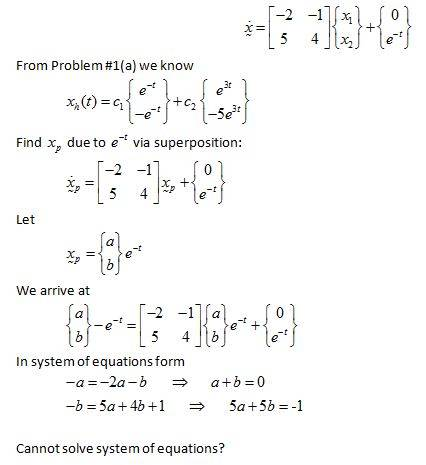# Cannot find nonhomogenous DE solution (should be easy?)

## Homework Statement

Determine the non-homogenous solution of the given differential equation.

See 3.

## The Attempt at a Solution

I have solved for the homogenous part, but as you can see in the link I am getting an unsolvable system of equations with the substitution method on the non-homogenous part. What am I doing wrong?## The Attempt at a Solution

ehild
Homework Helper
You have to choose an other trial function for xp, as the inhomogeneous part contains the same exponent as one of the exponents in the homogeneous part.

ehild

pasmith
Homework Helper

## Homework Statement

Determine the non-homogenous solution of the given differential equation.

See 3.

## The Attempt at a Solution

I have solved for the homogenous part, but as you can see in the link I am getting an unsolvable system of equations with the substitution method on the non-homogenous part. What am I doing wrong?Try
$$x_p = a\begin{pmatrix} 1 \\ -1 \end{pmatrix} te^{-t} + b\begin{pmatrix}1 \\ -5\end{pmatrix} e^{-t}$$